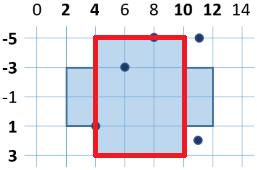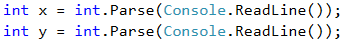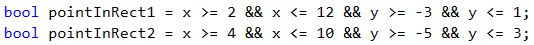# Problem: Point in a Figure

The next sample exam problem is about checking whether given point stays inside or outside given figure (see the image below).

## Video: Point in a Figure

Watch the video lesson about solving the "Point in a Figure" problem: https://youtu.be/SzxHyZVdhEo.

## Problem Description

Write a program that checks whether a point (with coordinates x and y) is inside or outside the following figure:## Input

The console reads two integers (one per line): x and y.

All input numbers are integers in the range [-1000 … 1000].

## Output

Print on the console "in" or "out" – whether the point is inside or outside the figure (the outline is inside).

## Sample Input and Output

Input Output Input Output
8
-5
in 6
-3
in
Input Output Input Output
11
-5
out 11
2
out

## Hints and Guidelines

To find out if the point is in the figure, we will divide the figure into 2 rectangles:A sufficient condition is the point to be located in one of them, in order to be in the figure.

## Determining the Point Location

We read the input data from the console:We will initialize two variables that will mark whether the point is in one of the rectangles.When printing the message, we will check whether any of the variables has accepted a value of true. It is enough only one of them to be true, so that the point is in the figure.## Testing in the Judge System

Test your solution here: https://judge.softuni.org/Contests/Practice/Index/516#3.